# Measure Line Segments Unlike a line a line

• Slides: 12Measure Line Segments Unlike a line, a line segment, or segment, can be measured because it has two endpoints. A segment with endpoints A and B can be named AB or BA. The length or measure of AB is written as AB A mm B 1 2 AB is about 28 millimeters long. 3 4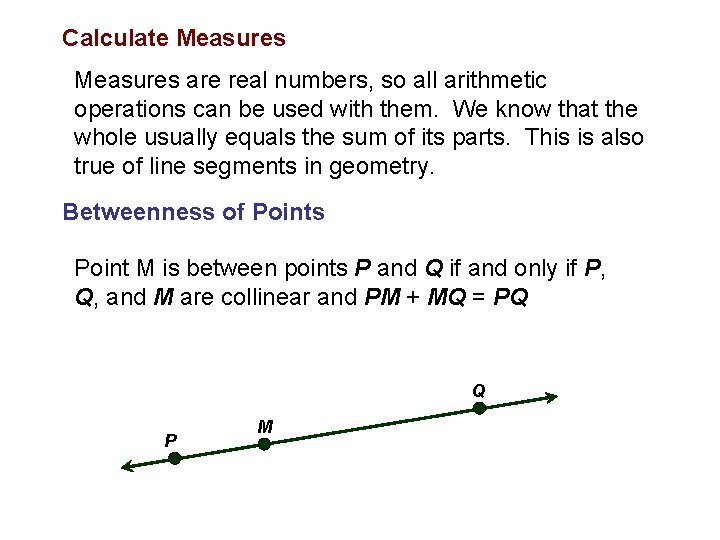Calculate Measures are real numbers, so all arithmetic operations can be used with them. We know that the whole usually equals the sum of its parts. This is also true of line segments in geometry. Betweenness of Points Point M is between points P and Q if and only if P, Q, and M are collinear and PM + MQ = PQ Q P MExample 1 - Find Measurements 1. Find AC AC is the measure of AC A 3. 3 cm B 3. 4 cm C AC can be found by adding AB and BC AB + BC = AC Sum of the parts = whole 3. 3 + 3. 4 = AC Substitution 6. 7 = AC Add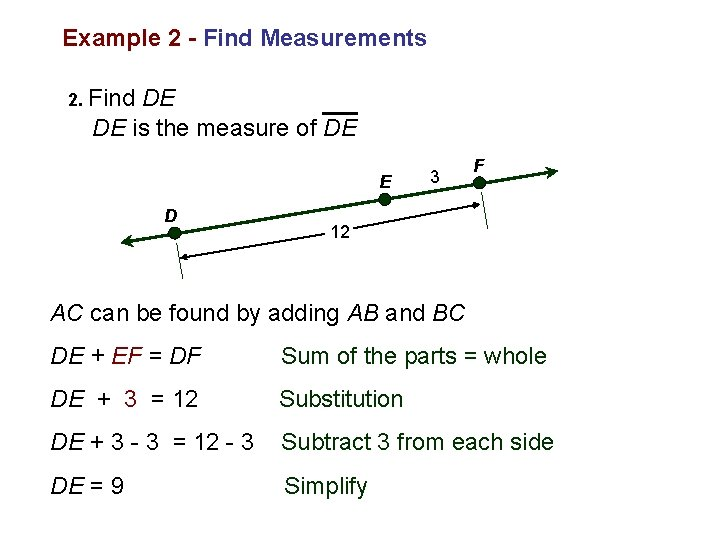Example 2 - Find Measurements 2. Find DE DE is the measure of DE E D 3 F 12 AC can be found by adding AB and BC DE + EF = DF Sum of the parts = whole DE + 3 = 12 Substitution DE + 3 - 3 = 12 - 3 Subtract 3 from each side DE = 9 SimplifyExample 3 - Find Measurements 3. Find y and PQ if P is between Q and R, PQ = 2 y, QR = 3 y + 1, and PR = 21 3 y + 1 21 2 y Q P R QR = QP + PR 3 y + 1 = 2 y + 21 3 y + 1 -1 = 2 y + 21 – 1 3 y = 2 y + 20 3 y – 2 y = 2 y + 20 – 2 y y = 20 Substitute known values Subtract one from each side Simplify Subtract 2 y from each side Simplify PQ = 2 y Given PQ = 2(20) y = 20 PQ = 40 multiply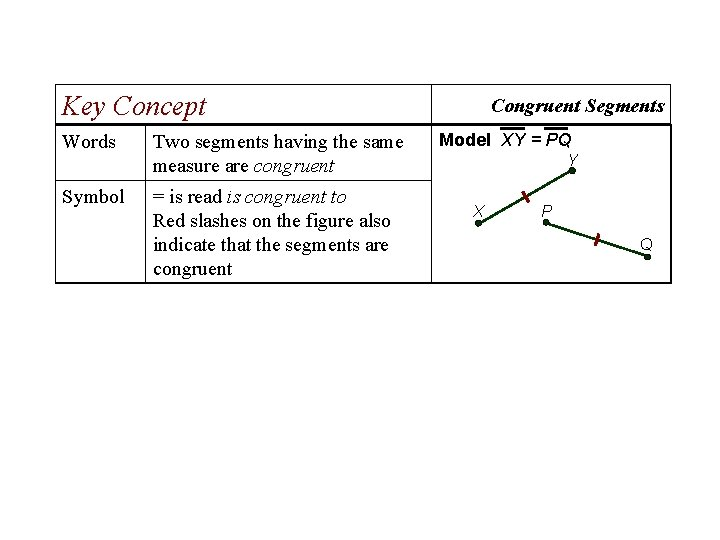Key Concept Words Two segments having the same measure are congruent Symbol = is read is congruent to Red slashes on the figure also indicate that the segments are congruent Congruent Segments Model XY = PQ Y X P QExample 4 - Find Measurements Find RS and ST 6 x - 5 R 2 x + 3 S 30 T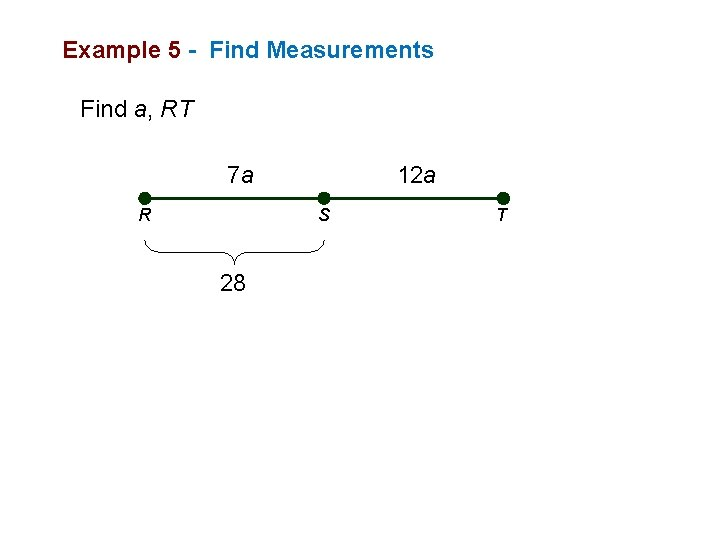Example 5 - Find Measurements Find a, RT 7 a R 12 a S 28 TExample 6 - Find Measurements Find x, RS and ST 2 x R 3 x S 25 T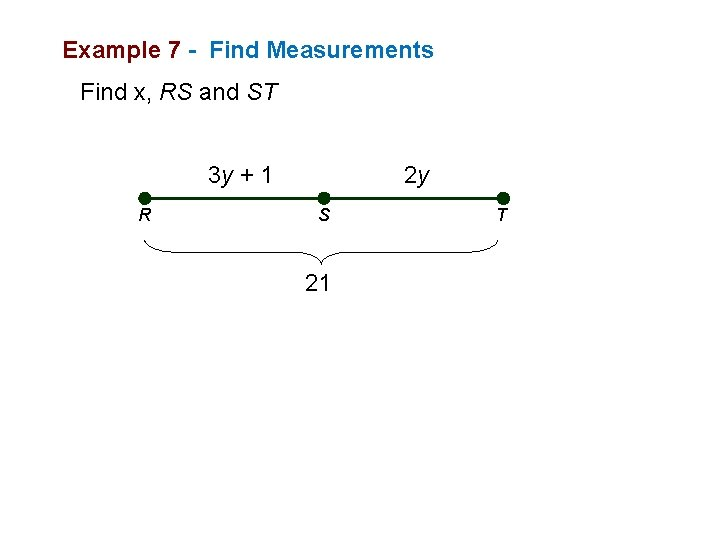Example 7 - Find Measurements Find x, RS and ST 3 y + 1 R 2 y S 21 TExample 8 - Find Measurements Find x, RT and ST 12 R 2 x S 5 x + 10 T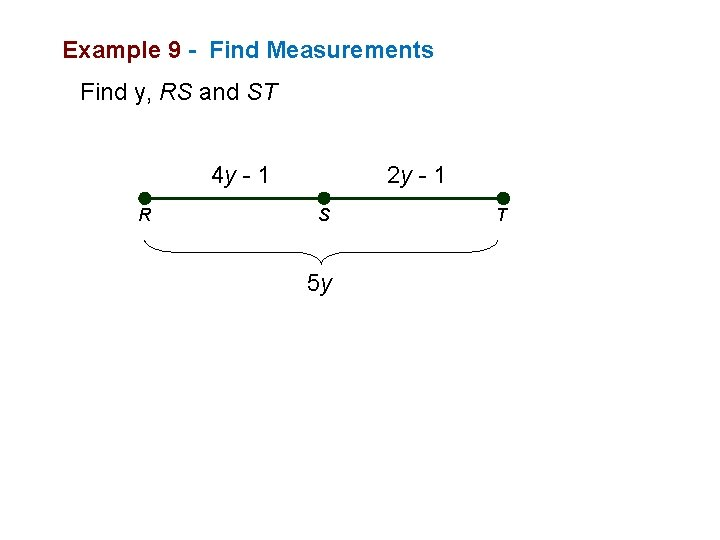Example 9 - Find Measurements Find y, RS and ST 4 y - 1 R 2 y - 1 S 5 y T